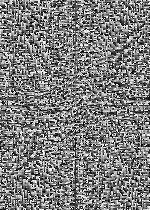## Some examples of Fourier approximation

The following are some pretty pictures to help visualize Fourier approximations, as discussed in Bill Faris' Math 511B (Real Analysis) course of spring 2006.

The context is real periodic functions on the interval from -π to π. For each of the eight functions below, the Fourier coefficients are estimated for n from -20 to 20:The Nth approximation isThese approximations are plotted for N from 0 to 20, along with the original function.

Last, the Fourier coefficents cn are plotted vs. n, for n from -20 to 20. Color scheme:

• For the function plots, the function is in blue.
• For the approximation plots, the original function is in blue, the constant-term approximation is in red, and then other colors are used for higher-order approximations.
• For the coefficient plots, real parts are in blue and imaginary parts are in red.

All the computations here are done numerically using the following Python script: four1. In this script, Fourier coefficients are estimated using naive quadrature (on a mesh of 400 points uniformly spaced from -π to π), as familiar from freshman calculus. Plots were made by piping the output from the four1 script to the xgr program.

John Kerl, 2006-03-06

Here is the piecewise "hat" function which has value 1 between -π/2 and π/2, and 0 outside (with period 2 π).

• Note that the constant term raises the level to 1/2. This is the average value of the function.
• Also note that the horns (these are called Gibbs phenomena in the engineering community) do not get appreciably lower (although they do get narrower) as |n| increases. This reflects the mathematical notion (as explained by Dr. Faris) that the convergence is in the zero-measure sense rather than in the pointwise sense.
• This is an even function, whereas cosine is even and sine is odd. Thus, as we expect, the Fourier coefficients here have imaginary parts (approximately, due to the numerical technique) equal to zero.

Function and approximations:Fourier coefficients:Function data, approximation data, coefficient data.

Here is the function f(x)=x (with period 2 π).

• This is an odd function, so here the Fourier coefficients have zero real part.
• The Fourier coefficients decay rather slowly to zero as |n| increases, as reflected in the homework assignment where we computed the coefficients analytically.
• Here too, the horns narrow but do not appreciably shorten as |n| increases.

Function and approximations: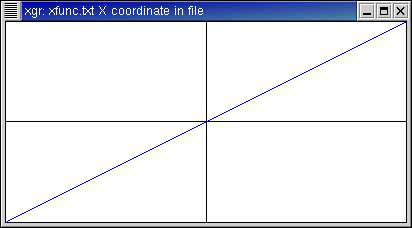Fourier coefficients: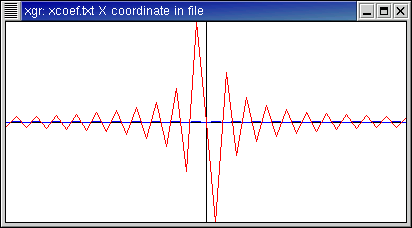Function data, approximation data, coefficient data.

Here is the function f(x)=π - |x| (with period 2 π).

• Like f(x)=x, this is not smooth. However it is continuous. Note that the Fourier coefficients go to zero more quickly than for the previous, discontinuous function.

Function and approximations: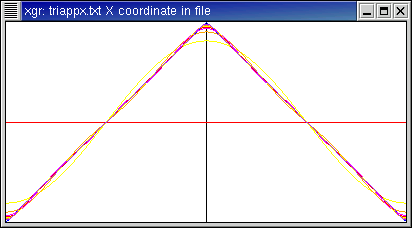Fourier coefficients: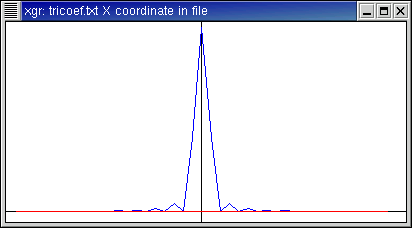Function data, approximation data, coefficient data.

Here is the function f(x)=1/(1+x2), with period 2 π.

• This could be made truly periodic using the Poisson formula. However the periodization would contribute little numerically, since f(x) is already nearly zero toward the edges.
• This is a smooth function (if written on the real line rather than on the circle ...), and the coefficients drop off rather quickly as seen in the coefficient plot.
• This can also be seen in the approximation plot, where the successive approximations get indistinguishably close to the original function after about the 5th term or so.

Function and approximations: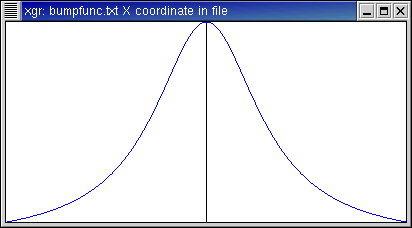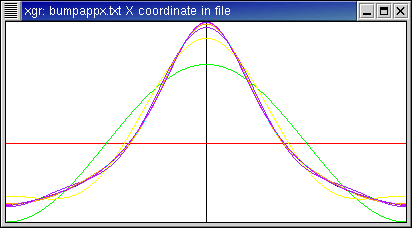Fourier coefficients: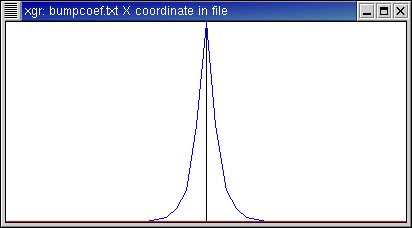Function data, approximation data, coefficient data.

Here is the function f(x)=cos(x).

• This function is exactly a cosine with integer frequency.
• The n=1 and n=-1 terms contribute all the energy, and reproduce the function exactly. The higher-order terms contribute nothing at all.
• The nth basis function is einx = cos(nx) + i sin(nx). Since cosine is even and sine is odd, for -n we have cos(-nx) + i sin(-nx) = cos(nx) - i sin(nx). Thus it takes the n=1 and the n=-1 terms, each with coefficient 1/2, to obtain the cosine part while canceling the sine part.

Function and approximations: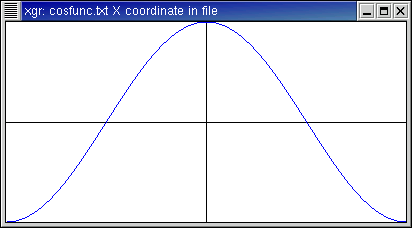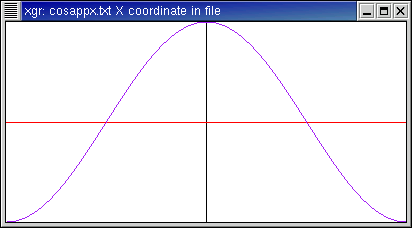Fourier coefficients:Function data, approximation data, coefficient data.

Here is the function f(x)=cos(1.7x+0.4).

• This function is a cosine, but with non-integer frequency.
• The coefficient plot shows most energy at |n| = 1 and |n| = 2, which makes sense since these two integer frequencies bracket the 1.7.
• The higher-order terms are non-zero.
• Note that the first few Fourier coefficients have appreciably non-zero imaginary part. This is because, while the cosine function is even, it is no longer even after the phase shift.
• Since the argument to cosine is 1.7x + 0.4, this function when written with period 2 π is not continuous. This fact is reflected by how the approximations behave near the edges.

Function and approximations: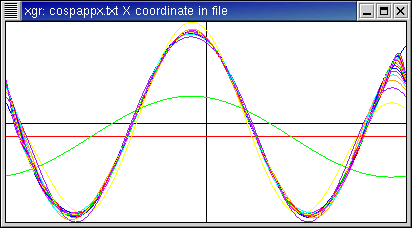Fourier coefficients: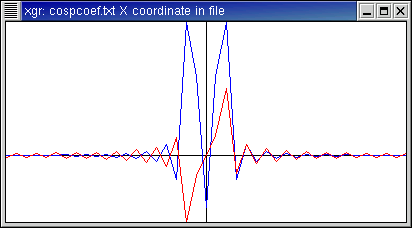Function data, approximation data, coefficient data.

Here is a function which (very simplistically) represents John, Paul, George, and Ringo doing the "LAHH" of the "lah-lah-lah-LAHH" from Twist and Shout. This is f(x)=cos(4x) + cos(5x) + cos(6x) + 1.2cos(7x).

• By the 7th term, the original function has been approximated exactly.
• These transforms are all discrete; I have done the plots with connected dots for visual convenience. In the coefficient plot, you see amplitudes 1/2, 1/2, 1/2, and 1.2/2 at n at 4, 5, 6, and 7, respectively. (The factors of 1/2 are for the same reason as the cos(x) example.) This provides visual confirmation of the linearity of the transform.

Function and approximations:Fourier coefficients:Function data, approximation data, coefficient data.

Here is the function f(x)=exp(-π x2), written with period 2 π.

• This is a Gaussian. Here we have visual confirmation that the transform of a Gaussian is another Gaussian.
• As with 1/(1+x2), periodization using the Poisson formula would contribute little numerically since the bell curve already falls off to zero near the edges.
• In general, the narrower the original Gaussian, the wider the transform and vice versa.
• The transform looks pointy. This is because the original function is plotted on a mesh of 400 points from x from -π to π, whereas I am only showing 41 Fourier coefficients, namely, for n from -20 to 20.

Function and approximations: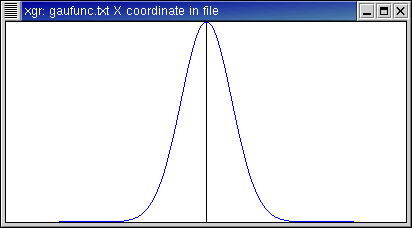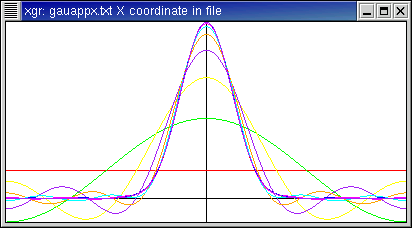Fourier coefficients:Function data, approximation data, coefficient data.

Here is a photo, right-clicked from Dr. Faris' web site, along with its 2D discrete Fourier transform.

Technical notes:

Mathematical notes:

• You can think of a 2D discrete Fourier transform as being on the torus, sampled with M points along rows and N points along columns.
• I put the zero frequencies at the center. Since the JPEG file contains values from 0 to 255, the image mean is certainly non-zero. That explains why the center spot is the brightest.
• The magnitude-of-transform plot was done using logarithm of complex magnitude of Fourier coefficients. This permits the high-frequency (off-center) components to still be seen. Otherwise, all but the center spot would appear black.
• For the phase plot, -π is black and π is white.
• Most of the transform energy is toward the center. This indicates that low-frequency components (such as facial features) are predominant. (The transform would be different if, say, we had a picture of a picket fence.)